﻿ GATE CS Applied Course

## GATE Previous Subject Wise Questions

##### Question 201
Consider a binary max-heap implemented using an array. Which one of the following array represents a binary max-heap?
A
25,12,16,13,10,8,14
B
25,14,13,16,10,8,12
C
25,14,16,13,10,8,12
D
25,14,12,13,10,8,16
Data Structures gate 2009 Heap-Tree
##### Question 202
Consider a binary max-heap implemented using an array. What is the content of the array after two delete operations on the correct answer to the previous question?
A
14,13,12,10,8
B
14,12,13,8,10
C
14,13,8,12,10
D
14,13,12,8,10
Data Structures gate 2009 Heap-Tree
##### Question 203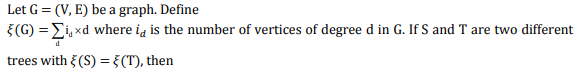A
| S | = 2 | T |
B
| S | = | T | - 1
C
| S | = | T |
D
| S | = | T | + 1

Mathematics Graph Theory gate 2010
##### Question 204
In a binary tree with n nodes, every node has an odd number of descendants. Every node is considered to be its own descendant. What is the number of nodes in the tree that have exactly one child?
A
0
B
1
C
(n-1)/2
D
n-1
Data Structures gate 2010 Binary-Trees
##### Question 205
Two alternative packages A and B are available for processing a database having 10k records.Package A requires 0.0001n2 time units and package B requires 10nlog10n time units to process n records. What is the smallest value of k for which package B will be preferred over A?
A
12
B
10
C
6
D
5
Algorithms Time-Complexity gate 2010
##### Question 206
The degree sequence of a simple graph is the sequence of the degrees of the nodes in the graph in decreasing order. Which of the following sequences can not be the degree sequence of any graph?
(I) 7, 6, 5, 4, 4, 3, 2, 1
(II) 6, 6, 6, 6, 3, 3, 2, 2
(III) 7, 6, 6, 4, 4, 3, 2, 2
(IV) 8, 7, 7, 6, 4, 2, 1, 1
A
I and II
B
III and IV
C
IV only
D
II and IV
Graph Theory gate 2010 Engineering-Mathematics
##### Question 207A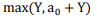B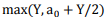C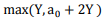DAlgorithms Dynamic-Programming gate 2010
##### Question 208
Let G be a group with 15 elements. Let L be a subgroup of G. It is known that L ≠ G and that the size of L is at least 4.  The size of L  is __________.
A
5
B
6
C
7
D
8
GATE 2014 SET 3
##### Question 209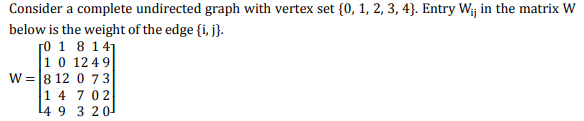What is the minimum possible weight of a spanning tree T in this graph such that vertex 0 is a leaf node in the tree T?
A
7
B
8
C
9
D
10
Algorithms Minimum-Spanning-Tree gate 2010
##### Question 210What is the minimum possible weight of a path P from vertex 1 to vertex 2 in this graph such that P contains at most 3 edges?
A
7
B
8
C
9
D
10
Data Structures gate 2010 Graphs
##### Question 211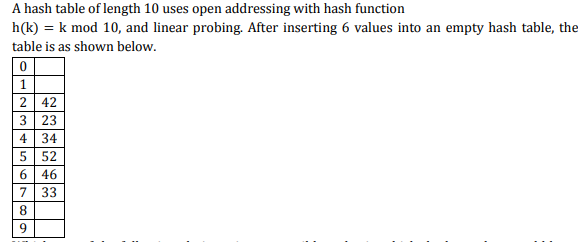Which one of the following choices gives a possible order in which the key values could have been inserted in the table?
A
46, 42, 34, 52, 23, 33
B
34, 42, 23, 52, 33, 46
C
46, 34, 42, 23, 52, 33
D
42, 46, 33, 23, 34, 52
Data Structures Hashing gate 2010
##### Question 212
Consider the following processors (ns stands for nanoseconds). Assume that the pipeline registers have zero latency. P1: Four-stage pipeline with stage latencies 1 ns, 2 ns, 2 ns, 1 ns. P2: Four-stage pipeline with stage latencies 1 ns, 1.5 ns, 1.5 ns, 1.5 ns. P3: Five-stage pipeline with stage latencies 0.5 ns, 1 ns, 1 ns, 0.6 ns, 1 ns. P4: Five-stage pipeline with stage latencies 0.5 ns, 0.5 ns, 1 ns, 1 ns, 1.1 ns. Which processor has the highest peak clock frequency?
A
P1
B
P2
C
P3
D
P4
computer organization GATE 2014 SET 3
##### Question 213
Consider the following rooted tree with the vertex la beled P as t he root:he order in which the nodes are visited during an i n-order trave rsal of the tree is
A
SQPTRWUV
B
SQPTUWRV
C
SQPTWUVR
D
SQPTRUWV
Algorithms GATE 2014 SET 3
##### Question 214
Suppose depth first search is executed on the graph below starting at some unknown vertex. Assume that a recursive call to visit a vertex is made only after first checking that the vertex has not been visited earlier. Then the maximum possible recursion depth (including the initial call) is _________.A
19
B
20
C
21
D
22
Algorithms GATE 2014 SET 3 Graphs
##### Question 215
You have an array of n elements. Suppose you implement quicksort by always choosing the central element of the array as the pivot. Then the tightest upper bound for the worst case performance is
A
O(n2)
B
O(n log n)
C
Θ(n log?n)
D
O(n3)
Algorithms Sorting GATE 2014 SET 3
##### Question 216
A system uses 3 page frames for storing process pages in main memory. It uses the Least Recently Used (LRU) page replacement policy. Assume that all the page frames are initially empty. What is the total number of page faults that will occur while processing the page reference string given below?   4, 7, 6, 1, 7, 6, 1, 2, 7, 2
A
6
B
7
C
8
D
9
operating systems GATE 2014 SET 3
##### Question 217
A system contains three programs and each requires three tape units for its operation. The minimum number of tape units which the system must have such that deadlocks never arise is _________.
A
7
B
8
C
9
D
10
operating systems GATE 2014 SET 3
##### Question 218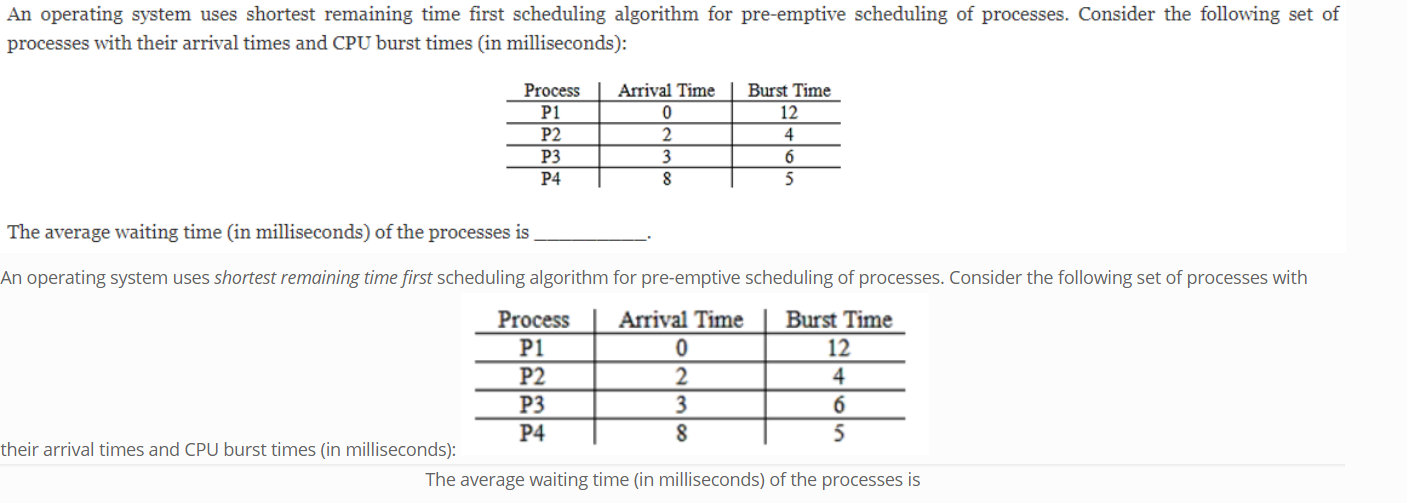A
5.5
B
5.6
C
5.7
D
5.8
operating systems GATE 2014 SET 3
##### Question 219
Consider a paging hardware with a TLB. Assume that the entire page table and all the pages are in the physical memory. It takes 10 milliseconds to search the TLB and 80 milliseconds to access the physical memory. If the TLB hit ratio is 0.6, the effective memory access time (in milliseconds) is  _________.
A
122
B
123
C
124
D
125
operating systems GATE 2014 SET 3
##### Question 220
Suppose you want to move from 0 to 100 on the number line. In each step, you either move right by a unit distance or you take a shortcut. A shortcut is simply a pre-specified pair of integers i, j with i < j.  Given a shortcut i, j if you are at position i on the number line, you may directly move to j. Suppose T(k) denotes the smallest number of steps needed to move from k to 100. Suppose further that there is at most 1 shortcut involving any number, and in particular from 9 there is a shortcut to 15. Let y and z be such that T(9) = 1 + min(T(y),T(z)).  Then the value of the product yz is _____.
A
150
B
151
C
 152
D
153
GATE 2014 SET 3© 2023 - All rights are reserved- AAIC Technologies pvt ltd